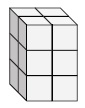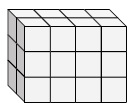• info@gomaths.co.za
• +27 (010) 312 6485

# Volume of a Rectangular Prism made of Unit Cubes

A unit cube has a length of 1 unit, width of 1 unit and height of 1 unit. Its volume is 1 cubic unit.

Any solid shape can be shown as being of made of unit cubes. Counting the number of unit cubes in that solid shape gives its volume. The cube shaded green is a unit cube.Definition of volume: The number or amount of cubic units it takes to fill a defined space is called volume.

## Formula for the volume of a rectangular prism

The volume of a rectangular prism of length l, width w and height h is given by the formula.

The volume of a rectangular prism is given by the product of length, width and the height h of the prism.

Volume V = l × w × h cubic units

Find the length, width and height of the following rectangular prism. Then find its volume.### Solution

Step 1:

The given prism has length = 2; width = 3; height = 2

Step 2:

The volume of prism V = l × w × h

= 2 × 3 × 2

= 12 cubic units.

Find the length, width and height of the following rectangular prism. Then find its volume.### Solution

Step 1:

The given prism has length = 4; width = 3; height = 2

Step 2:

The volume of prism V = l × w × h

= 4 × 3 × 2

= 24 cubic units.

Back to: 3d Figures and Volumes > Chapter 2: Case aeque melius duo ut, porro ridens habemus quo in. (Marc Twain)